Question

# You have 659.3 mL of a stock solution 4.660 M HCl. For a particular experiment, your...

You have 659.3 mL of a stock solution 4.660 M HCl. For a particular experiment, your lab partner prepared a diluted solution for you to use. In her notes, you read: " Using a volumetric pipet, took 246.54 mL of stock solution and diluted it to 739.21 ml in a volumetric flask. Then, took 100.00 mL of that solution and diluted it to 194.63 mL in a second volumetric flask. " What is the concentration that you should report for your experiment?

First of all we know about stock solution that is a solution of known concentration ,here we do the process as dilution and here use for mulae is M1V1 =M2V2 in first case and in second case as M2V2=M3V3.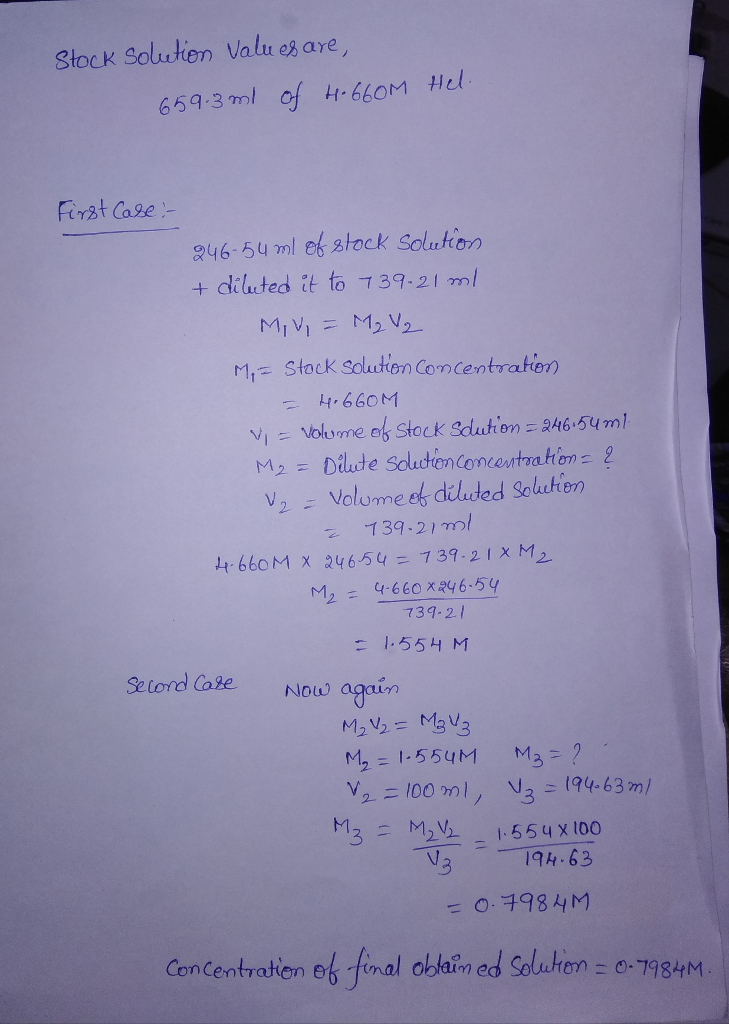#### Earn Coins

Coins can be redeemed for fabulous gifts.

Similar Homework Help Questions
• ### You prepared a set of standard solutions by serial dilutions. You started with a stock solution...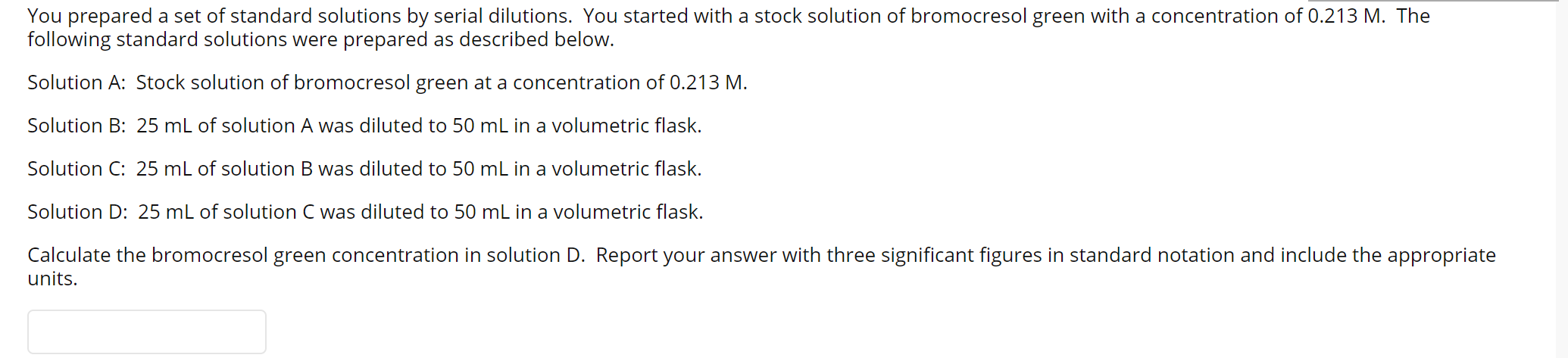You prepared a set of standard solutions by serial dilutions. You started with a stock solution of bromocresol green with a concentration of 0.213 M. The following standard solutions were prepared as described below. Solution A: Stock solution of bromocresol green at a concentration of 0.213 M. Solution B: 25 mL of solution A was diluted to 50 mL in a volumetric flask. Solution C: 25 mL of solution B was diluted to 50 mL in a volumetric flask. Solution...

• ### Suppose you have 100.00 mL of a solution of a dye and transfer 2.00 mL of...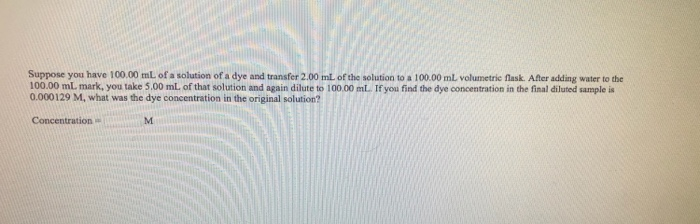Suppose you have 100.00 mL of a solution of a dye and transfer 2.00 mL of the solution to a 100.00 mL volumetric flask. After adding water to the 100.00 mL mark, you take 5.00 mL of that solution and again dilute to 100.00 mL. If you find the dye concentration in the final diluted sample is 0.000129 M, what was the dye concentration in the original solution? Concentration

• ### Stock solutions are HCl and NaOH If you are given the following solids: NasPO4 K3PO4 NAH2PO4...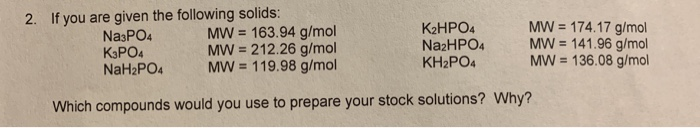Stock solutions are HCl and NaOH If you are given the following solids: NasPO4 K3PO4 NAH2PO4 2. MW 174.17 g/mol MW= 141.96 g/mol MW- 136.08 g/mol K2HPO4 NazHPO4 KH2PO4 MW = 163.94 g/mol MW 212.26 g/mol MW 119.98 g/mol Which compounds would you use to prepare your stock solutions? Why? given deionized water, the solid you selected in the previous question and the following If you are volumetric glassware: 100.00 ml volumetric flask(s) 250.00 ml volumetric flask(s) 25.00 ml volumetric...

• ### You must read the experiment and then answer these questions. 1. Look at the procedure for...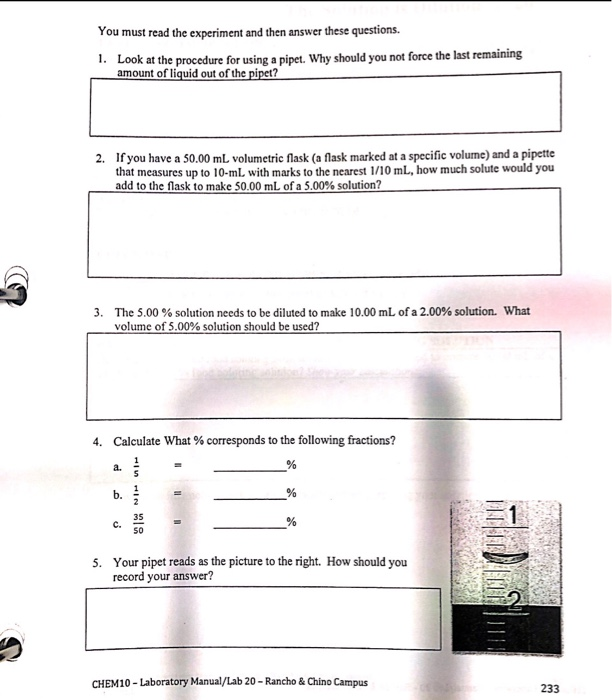You must read the experiment and then answer these questions. 1. Look at the procedure for using a pipet. Why should you not force the last remaining amount of liquid out of the pipet? 2. If you have a 50.00 mL volumetric flask (a flask marked at a specific volume) and a pipette that measures up to 10-mL with marks to the nearest 1/10 mL, how much solute would you add to the flask to make 50.00 mL of a...

• ### Question 1 2 pts 3.34 mL of an unknown glucose solution was diluted in a 100.00 mL of volumetric flask with distill...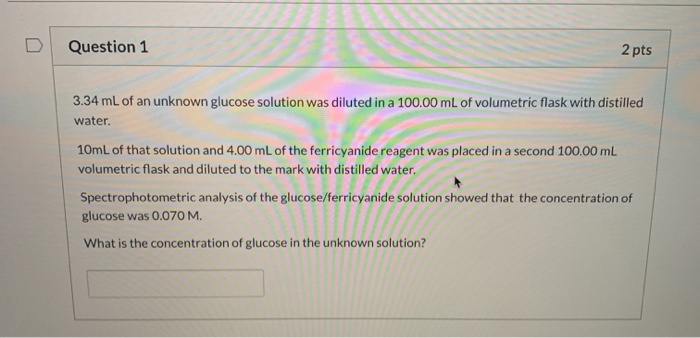Question 1 2 pts 3.34 mL of an unknown glucose solution was diluted in a 100.00 mL of volumetric flask with distilled water. 10mL of that solution and 4.00 mL of the ferricyanide reagent was placed in a second 100.00 mL volumetric flask and diluted to the mark with distilled water. Spectrophotometric analysis of the glucose/ferricyanide solution showed that the concentration of glucose was 0.070 M. What is the concentration of glucose in the unknown solution?

• ### Question 1 2 pts 3.34 mL of an unknown glucose solution was diluted in a 100.00 mL of volumetric flask with distill...Question 1 2 pts 3.34 mL of an unknown glucose solution was diluted in a 100.00 mL of volumetric flask with distilled water. 10mL of that solution and 4.00 mL of the ferricyanide reagent was placed in a second 100.00 mL volumetric flask and diluted to the mark with distilled water. Spectrophotometric analysis of the glucose/ferricyanide solution showed that the concentration of glucose was 0.070 M. What is the concentration of glucose in the unknown solution?

• ### Calculate the concentration of the standard HCl solution you prepared. Determine this concentration for each trial...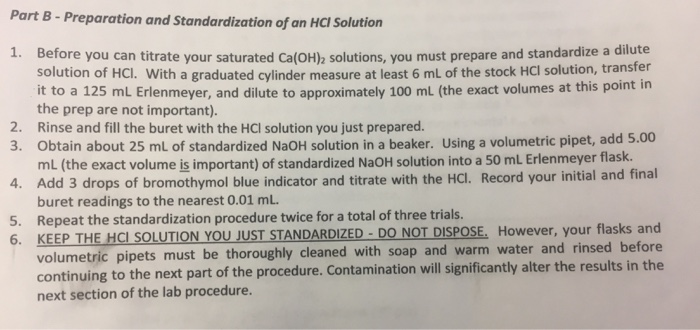Calculate the concentration of the standard HCl solution you prepared. Determine this concentration for each trial and the average and standard deviation for all three trials. Part B-Preparation and standardization of an HCl Solution 1. Before you can titrate your saturated Ca(oH)a solutions, you must prepare and standardize a dilute solution of Hcl. With a graduated cylinder measure at least 6 mL of the stock Hcl lution, transfer it to a 125 mL Erlenmeyer, and dilute to approximately 100 mL...

• ### Electrolytes, Concentration and Dilution of Solutions n this part of the experiment, you will make a dilution of a stock solution of known concentration and calculate the concentration of the di...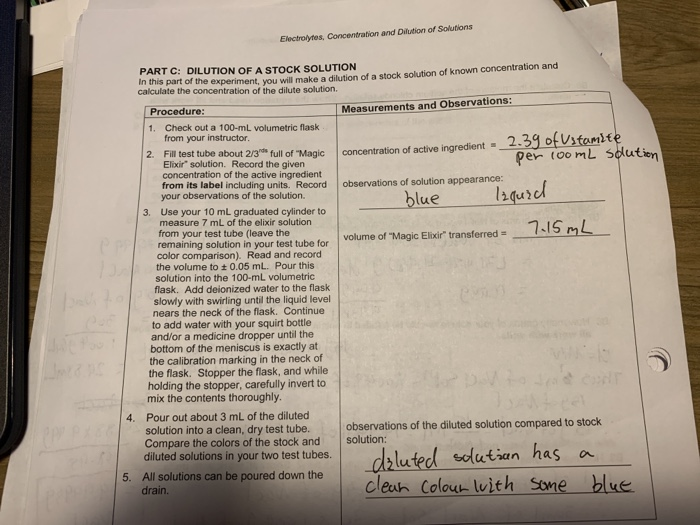Electrolytes, Concentration and Dilution of Solutions n this part of the experiment, you will make a dilution of a stock solution of known concentration and calculate the concentration of the dilute solution. DILUTION OF A STOCK SOLUTION PART C: Measurements and Observations Procedure Check out a 100-mL volumetric flask from your instructor. Fill test tube about 2/3 concentration of the active ingredient from its label including units. Record 1. orlt O " concentration of active ingredient. full of "Magic 2....

• ### A 2.0 M stock solution of Tylenol was diluted as follows: 15.000 mL of stock solution...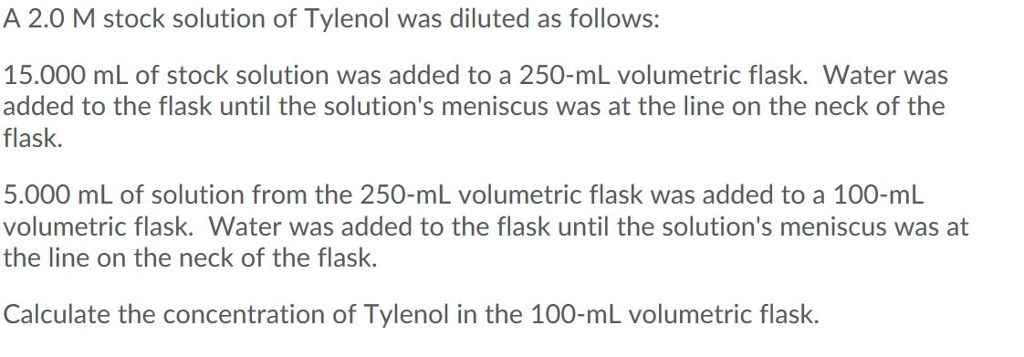A 2.0 M stock solution of Tylenol was diluted as follows: 15.000 mL of stock solution was added to a 250-ml volumetric flask. Water was added to the flask until the solution's meniscus was at the line on the neck of the flask. 5.000 mL of solution from the 250-ml volumetric flask was added to a 100-ml volumetric flask. Water was added to the flask until the solution's meniscus was at the line on the neck of the flask. Calculate...

• ### PRE-LAR STUY GESTIONS The Solution is Dilution LA 20 You must read the experiment and then...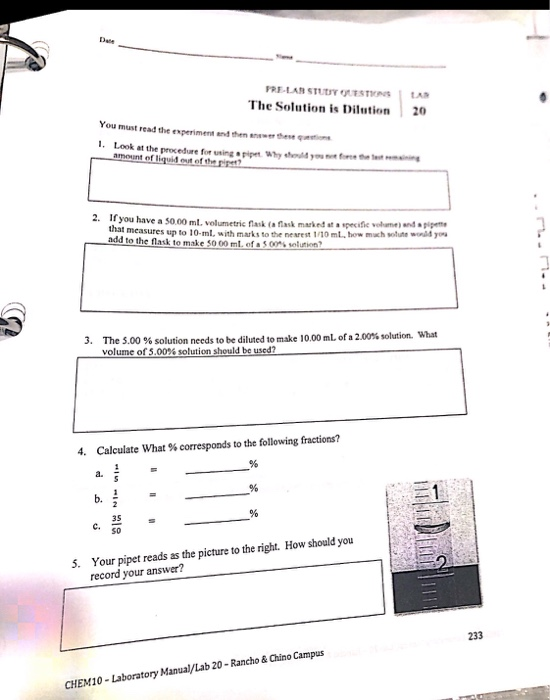PRE-LAR STUY GESTIONS The Solution is Dilution LA 20 You must read the experiment and then werde n 1. Look at the procedure for amount of liquit of the y o u 2. If you have a 50.00 ml. volumetric raskask a ta specife womendappe that measures up to 10-ml, with marks to the nearest 1/10 ml. how much more word you add to the flask to make 5000 mlofaS005 solution? 3. The 3,00 % solution needs to be diluted...